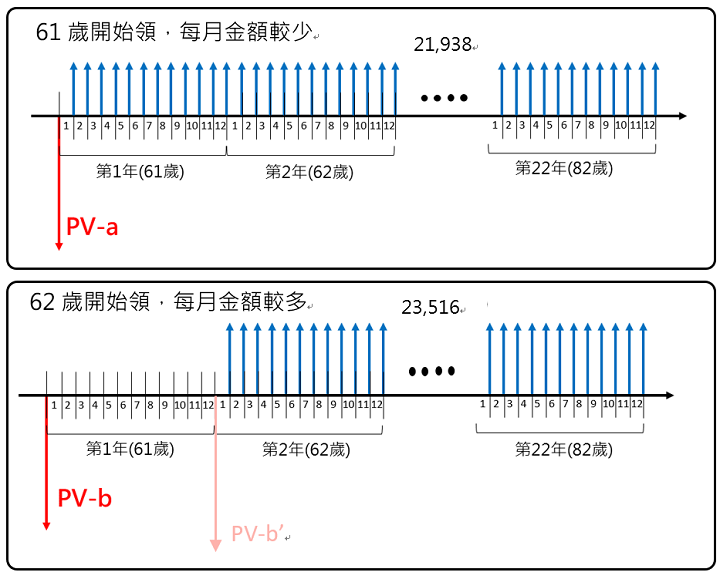# 勞保老年年金愈晚領愈划算

﻿=(1+1.3%)^(1/12)-1

61歲請領的年金現值PV-a：

=-PV((1+1.3%)^(1/12)-1, 22*12, 21938) = 503萬8,758元

=-PV((1+1.3%)^(1/12)-1, 21*12, 23516)

PV-b
= PV-b’/(1+1.3%)^1
= -PV((1+1.3%)^(1/12)-1, 21*12, 23516) /(1+1.3%)^1
= 512萬969元

## 附註：試算表現值公式

=-PV((1+投資報酬率)^(1/12)-1,[@領到年數]*12,[@月領金額])/(1+投資報酬率)^(COUNT(\$A\$9:A9)-1)

﻿

■ 本網站內容儘可能精確完整，但不保證無誤。若做為投資依據，風險請自行斟酌 ，本網站不負賠償之責任。
■ 網站所有資料均為版權所有，非經書面允許請勿轉載或使用。